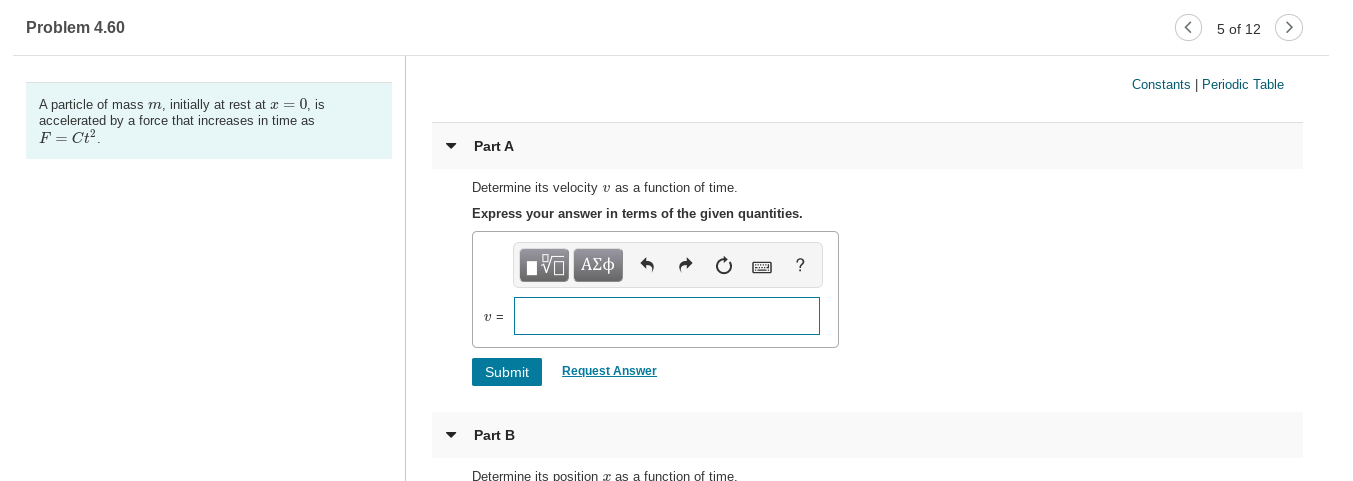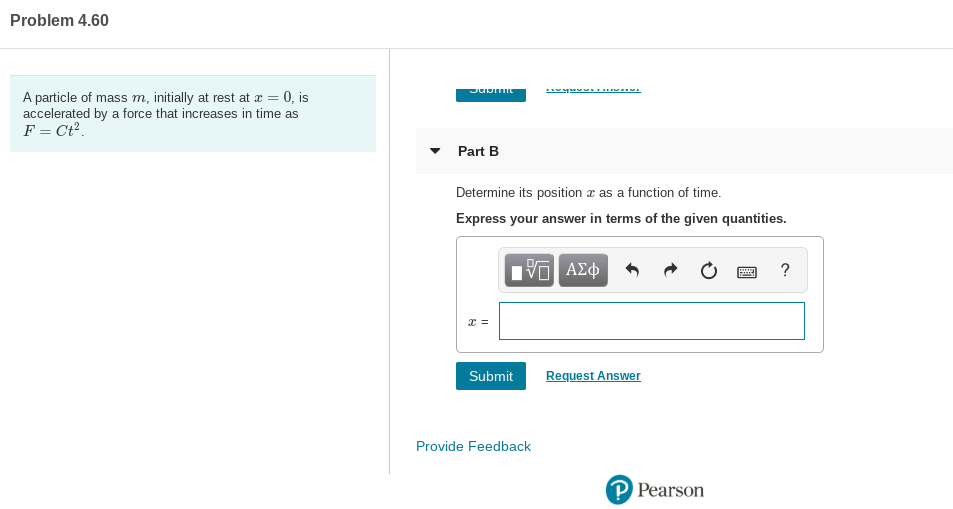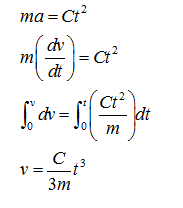# Problem 4.605 of 12Constants | Periodic TableA particle of mass m, initially at rest at æ = 0, isaccelerated by a force that increases in time asF = Ct?.Part ADetermine its velocity v as a function of time.Express your answer in terms of the given quantities.nν ΑΣφRequest AnswerSubmitPart BDetermine its position a as a function of time. Problem 4.60A particle of mass m, initially at rest at æ = 0, isaccelerated by a force that increases in time asF = Ct?.%3D• Part BDetermine its position z as a function of time.Express your answer in terms of the given quantities.V ΑΣφRequest AnswerSubmitProvide FeedbackP Pearson

Question
2 views

Please help me with all the parts, there are 2 total parts attached as 2 different screenshots.help_outlineImage TranscriptioncloseProblem 4.60 5 of 12 Constants | Periodic Table A particle of mass m, initially at rest at æ = 0, is accelerated by a force that increases in time as F = Ct?. Part A Determine its velocity v as a function of time. Express your answer in terms of the given quantities. nν ΑΣφ Request Answer Submit Part B Determine its position a as a function of time. fullscreenhelp_outlineImage TranscriptioncloseProblem 4.60 A particle of mass m, initially at rest at æ = 0, is accelerated by a force that increases in time as F = Ct?. %3D • Part B Determine its position z as a function of time. Express your answer in terms of the given quantities. V ΑΣφ Request Answer Submit Provide Feedback P Pearson fullscreen
check_circle

Step 1

a)

The expression for the velocity is,...

### Want to see the full answer?

See Solution

#### Want to see this answer and more?

Solutions are written by subject experts who are available 24/7. Questions are typically answered within 1 hour.*

See Solution
*Response times may vary by subject and question.
Tagged in

### Physics